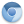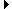Chromium Code Reviews
(8)

## Issue 159885: linux: yet more polish for the new toolbar graphics (Closed)

Created:
10 years, 9 months ago by Evan Martin
Modified:
9 years ago
CC:
Visibility:
Public.

### Description

linux: yet more polish for the new toolbar graphics

### Patch Set 1 #

Unified diffs Side-by-side diffs Delta from patch set Stats (+14 lines, -2 lines) PatchM app/resources/app_resources.grd View 1 chunk +2 lines, -0 lines 0 comments DownloadM chrome/app/generated_resources.grd View 1 chunk +3 lines, -0 lines 0 comments DownloadM chrome/browser/gtk/browser_titlebar.cc View 1 chunk +9 lines, -2 lines 0 comments Download

### Messages

Total messages: 2 (0 generated)
Evan Martin 10 years, 9 months ago (2009-08-04 23:47:19 UTC) #1
 Looks Glen To Me. -Ben On Tue, Aug 4, 2009 at 4:47 PM, wrote: ...
10 years, 9 months ago (2009-08-04 23:51:51 UTC) #2
```Looks Glen To Me.

-Ben

On Tue, Aug 4, 2009 at 4:47 PM, <evan@chromium.org> wrote:
> Reviewers: Ben Goodger,
>
> Description:
> linux: yet more polish for the new toolbar graphics
>
> Please review this at http://codereview.chromium.org/159885
>
> Affected files:
> =A0M app/resources/app_resources.grd
> =A0M chrome/app/generated_resources.grd
> =A0M chrome/browser/gtk/browser_titlebar.cc
>
>
> Index: app/resources/app_resources.grd
> diff --git a/app/resources/app_resources.grd
> b/app/resources/app_resources.grd
> index
> 8b7579dcaf3b71fd94165fe0cfbdd65760d0cddc..ee0c5a2f70e2d1f571e2c12227d77bf=
d9b74c4cf
> 100644
> --- a/app/resources/app_resources.grd
> +++ b/app/resources/app_resources.grd
> @@ -106,6 +106,8 @@
>
> =A0 =A0 =A0 <!-- Menus -->
ng"
> type=3D"BINDATA" />
> +
> + =A0 =A0 =A0<include name=3D"IDR_GLEN" file=3D"linux_close_glen.png" typ=
e=3D"BINDATA"
> />
> =A0 =A0 </includes>
> =A0 </release>
> =A0</grit>
> Index: chrome/app/generated_resources.grd
> diff --git a/chrome/app/generated_resources.grd
> b/chrome/app/generated_resources.grd
> index
> f6a0983b3146267d093d5d9733b898d2d3bb096f..1bb9f40b73c3708d6ba6cd124417c8d=
ef5311df8
> 100644
> --- a/chrome/app/generated_resources.grd
> +++ b/chrome/app/generated_resources.grd
> @@ -4621,6 +4621,9 @@ each locale. -->
> =A0 =A0 =A0 Open Files
> =A0 =A0 </message>
>
> + =A0 =A0<message translateable=3D"false" name=3D"IDS_GLEN" desc=3D"Glen"=
>
> + =A0 =A0 =A0Glen
> + =A0 =A0</message>
> =A0 =A0 </messages>
> =A0 </release>
> =A0</grit>
> Index: chrome/browser/gtk/browser_titlebar.cc
> diff --git a/chrome/browser/gtk/browser_titlebar.cc
> b/chrome/browser/gtk/browser_titlebar.cc
> index
> 11d34996ecbf57855c03477d3de5a072865b30a8..05520d007c74f21e4f08a24897d4a40=
00292c4d4
> 100644
> --- a/chrome/browser/gtk/browser_titlebar.cc
> +++ b/chrome/browser/gtk/browser_titlebar.cc
> @@ -191,8 +191,15 @@ void BrowserTitlebar::Init() {
> =A0 gtk_box_pack_start(GTK_BOX(titlebar_buttons_box_), buttons_hbox, FALS=
E,
> =A0 =A0 =A0 =A0 =A0 =A0 =A0 =A0 =A0 =A0 =A0FALSE, 0);
>
> - =A0close_button_.reset(BuildTitlebarButton(IDR_CLOSE, IDR_CLOSE_P,
> IDR_CLOSE_H,
> - =A0 =A0 =A0 =A0 =A0 =A0 =A0 =A0 =A0 =A0 =A0buttons_hbox, IDS_XPFRAME_CL=
OSE_TOOLTIP));
> + =A0if (CommandLine::ForCurrentProcess()->HasSwitch(L"glen")) {
> + =A0 =A0close_button_.reset(BuildTitlebarButton(IDR_GLEN, IDR_GLEN, IDR_=
GLEN,
> + =A0 =A0 =A0 =A0 =A0 =A0 =A0 =A0 =A0 =A0 =A0 =A0 =A0 =A0 =A0 =A0 =A0 =A0=
=A0 =A0 =A0 =A0buttons_hbox, IDS_GLEN));
> + =A0} else {
> + =A0 =A0close_button_.reset(BuildTitlebarButton(IDR_CLOSE, IDR_CLOSE_P,
> IDR_CLOSE_H,
> + =A0 =A0 =A0 =A0 =A0 =A0 =A0 =A0 =A0 =A0 =A0 =A0 =A0 =A0 =A0 =A0 =A0 =A0=
=A0 =A0 =A0 =A0buttons_hbox,
> + =A0 =A0 =A0 =A0 =A0 =A0 =A0 =A0 =A0 =A0 =A0 =A0 =A0 =A0 =A0 =A0 =A0 =A0=
=A0 =A0 =A0 =A0IDS_XPFRAME_CLOSE_TOOLTIP));
> + =A0}
> +
> =A0 restore_button_.reset(BuildTitlebarButton(IDR_RESTORE, IDR_RESTORE_P,
> =A0 =A0 =A0 =A0 =A0 =A0 =A0 =A0 =A0 =A0 =A0 =A0 IDR_RESTORE_H, buttons_hb=
ox,
> =A0 =A0 =A0 =A0 =A0 =A0 =A0 =A0 =A0 =A0 =A0 =A0 IDS_XPFRAME_RESTORE_TOOLT=
IP));
>
>
>```

This is Rietveld 408576698